# MP Board Class 8th Maths Solutions Chapter 1 Rational Numbers Ex 1.1

In this article, we will share MP Board Class 8th Maths Solutions Chapter 1 Rational Numbers Ex 1.1 Pdf, These solutions are solved subject experts from the latest edition books.

## MP Board Class 8th Maths Solutions Chapter 1 Rational Numbers Ex 1.1

Question 1.
Using appropriate properties, find.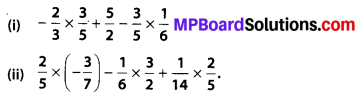Solution:Question 2.
Write the additive inverse of each of the followingSolution: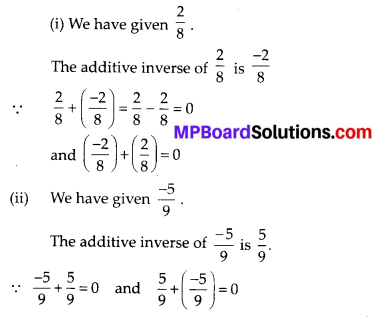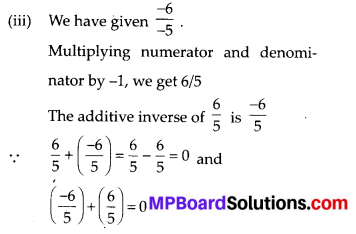(iv) We have given $$\frac{2}{-9}$$
Multiplying numerator and denominator by -1, we get $$\frac{2}{-9}$$
The additive inverse of $$\frac{2}{-9}$$ is $$\frac{2}{9}$$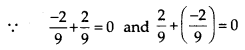(v) We have $$\frac{19}{-6}$$
Multiplying numerator and denominator by -1, we get $$\frac{-19}{6}$$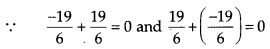Question 3.
Verify that -(-x) = x for
(i) x = $$\frac{11}{15}$$
(ii) x = $$-\frac{13}{17}$$
Solution:Question 4.
Find the multiplicative inverse of the following.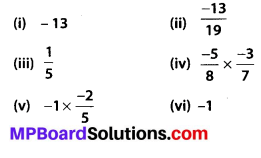Solution:
(i) We have given, -13
The multiplicative inverse of -13 is $$\left(\frac{-1}{13}\right)$$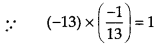(ii) We have given, $$\frac{-13}{19}$$
The multiplicative inverse of is $$\frac{-13}{19}$$ is $$\frac{-19}{13}$$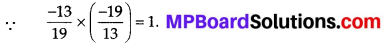(iii) We have given, $$\frac{1}{5}$$
The multiplicative inverse of $$\frac{1}{5}$$ is 5.
∵ $$\frac{1}{5} \times 5=1$$

(iv) We have given, $$\frac{-5}{8} \times \frac{-3}{7}$$
The multiplicative inverse of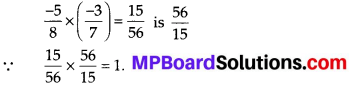(v) We have given, $$-1 \times \frac{-2}{5}$$
The multiplicative inverse of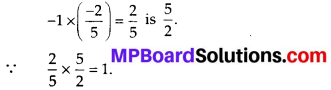(vi) We have given, -1.
The multiplicative inverse of -1 is -1.
∵ (-1) × (-1) = 1.

Question 5.
Name the property under multiplication used in each of the following.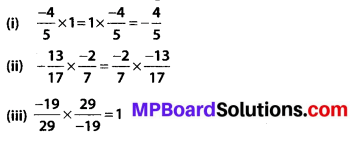Solution:
(i) We have given, $$\frac{-4}{5} \times 1=1 \times \frac{-4}{5}=\frac{-4}{5}$$
i.e., 1 is the multiplicative identity. Thus, it is a identity property under multiplication
(ii) We have given, $$\frac{-13}{17} \times \frac{-2}{7}=\frac{-2}{7} \times \frac{-13}{17}$$, which shows the commutativity.
Thus, it is a commutative property under multiplication.
(iii) We have given $$\frac{-19}{29} \times \frac{29}{-19}=1$$, which shows that $$\frac{29}{-19}$$ is a multiplicative inverse of $$\left(\frac{-19}{29}\right)$$
Thus, it is a inverse property under multiplication.Question 6.
Multiply $$\frac{6}{13}$$ by the reciprocal of $$\frac{-7}{16}$$.
Solution:Question 7.
Tell what property allows you to compute $$\frac{1}{3} \times\left(6 \times \frac{4}{3}\right) \text { as }\left(\frac{1}{3} \times 6\right) \times \frac{4}{3}$$
Solution:Above property is associativity.
[ ∵ a × (b × c) = (a × b) × c]Question 8.
Is $$\frac{8}{9}$$ the multiplicative inverse of $$-1 \frac{1}{8}$$ ? Why or why not?
Solution:
We have given a fraction $$\frac{8}{9}$$ and $$-1 \frac{1}{8}=\frac{-9}{8}$$
No, $$\frac{-9}{8}$$ is not a multiplicative inverse of $$\frac{8}{9}$$ because $$\frac{8}{9} \times\left(\frac{-9}{8}\right)=-1 \neq 1$$

Question 9.
Is 0.3 the multiplicative inverse of $$3 \frac{1}{3}$$ ? Why or why not?
Solution:Factoring simplifying rational expressions calculator.

Question 10.
Write.
(i) The rational number that does not have a reciprocal.
(ii) The rational numbers that are equal to their reciprocals.
(iii) The rational number that is equal to its negative.
Solution:
(i) 0 is the rational number, which does not have a reciprocal.
(ii) 1 and (-1) are the rational numbers, that are equal to their reciprocals.
(iii) 0 is the rational number that is equal to its negative.Question 11.
Fill in the blanks.
(i) Zero has …… reciprocal.
(ii) The numbers …… and ……. are their own reciprocals.
(iii) The reciprocals of -5 is ……
(iv) Reciprocal of $$\frac{1}{x}$$, where x ≠ 0 is ……
(v) The product of two rational numbers is always a ……
(vi) The reciprocal of positive rational number is …….
Solution:
(i) Zero has no reciprocal.
(ii) The numbers 1 and -1 are their own reciprocals.
(iii) The reciprocal of -5 is $$\frac{-1}{5}$$ .
(iv) Reciprocal of $$\frac{1}{x}$$, where x ≠ 0 is x.
(v) The product of two rational numbers is always a rational number.
(vi) The reciprocal of positive rational number is positive.